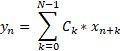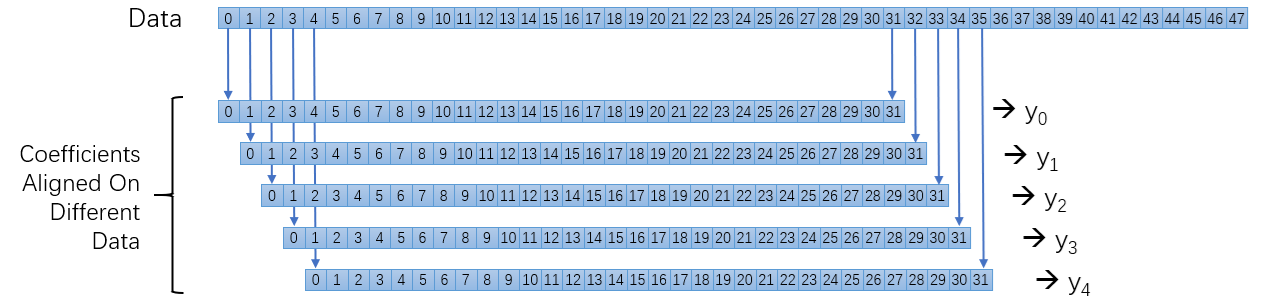# Multiple Kernels Coding Example: FIR Filter - 2022.2 English

## AI Engine Kernel and Graph Programming Guide (UG1079)

Document ID
UG1079
Release Date
2022-10-19
Version
2022.2 English

In this section, the filter design is used to demonstrate how to split the application into multiple AI Engines when an application exceeds the computational capacity of a single AI Engine. A finite impulse response (FIR) filter is a filter whose impulse response (or response to any finite length input) is of finite duration.Note: Mathematically, this equation is a correlation instead of a convolution. This is the way computation is organized within the AI Engine. For this to become a convolution (FIR filtering), the coefficients have to be stored in vector CK in the reverse order. This is not a problem for symmetric filters.

In the previous equation, N denotes the taps to be used to calculate each output. The calculation process when a 32 taps filter is used as an example is shown in the following figure. int16 complex types for data and coefficient are also used as an example.

Figure 1. FIR Filter